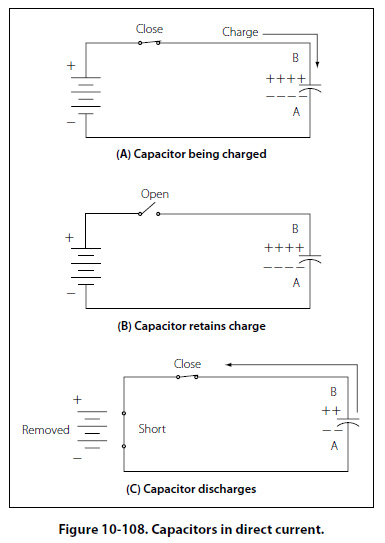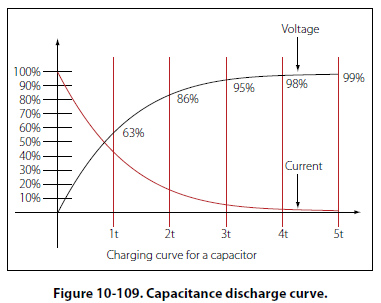Capacitance Another important property in AC circuits, besides resistance and inductance, is capacitance. While inductance is represented in a circuit by a coil, capacitance is represented by a capacitor. In its most basic form the capacitor is constructed of two parallel plates separated by a nonconductor, called a dielectric. In an electrical circuit, a capacitor serves as a reservoir or storehouse for electricity. Capacitors in Direct Current When a capacitor is connected across a source of direct current, such as a storage battery in the circuit shown in Figure 10-108A, and the switch is then closed, the plate marked B becomes positively charged, and the A plate negatively charged. Current flows in the external circuit during the time the electrons are moving from B to A. The current flow in the circuit is at a maximumthe instant the switch is closed, but continually decreases thereafter until it reaches zero. The current becomes zero as soon as the difference in voltage of A and B becomes the same as the voltage of the battery. If the switch is opened as shown in Figure 10-108B, the plates remain charged. Once the capacitor is shorted, it will discharge quickly as shown Figure 10-108C. It should be clear that during the time the capacitor is being charged or discharged, there is current in the circuit, even though the circuit is broken by the gap between the capacitor plates. Current is present only during the time of charge and discharge, and this period of time is usually short. The RC Time Constant The time required for a capacitor to attain a full charge is proportional to the capacitance and the resistance of the circuit. The resistance of the circuit introduces the element of time into the charging and discharging of a capacitor. When a capacitior charges or discharges through a resistance, a certain amount of time is required for a full charge or discharge. The voltage across the capacitor will not change instantaneously. The rate of charging or discharging is determined by the time constant of the circuit. The time constant of a series RC (resistor/ capacitor) circuit is a time interval that equals the product of the resistance in ohms and the capacitance in farad and is symbolized by the greek letter tau (τ). τ = RC The time in the formula is that required to charge to 63% of the voltage of the source. The time required to bring the charge to about 99% of the source voltage is approximately 5 t. Figure 10-109 illustrates this relationship of a time constant characteristics of charging.The measure of a capacitor’s ability to store charge is its capacitance. The symbol used for capacitance is the letter C. As can be seen from the time constant illustration there can be no continuous movement of direct current through a capacitor. A good capacitor will block direct current and will pass the effects of pulsing DC or alternating current.
 ©AvStop Online Magazine                                                                                                                                                      Contact Us              Return To Books